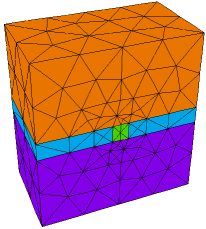﻿ Sample Problems > Usage > 3D_domains > 3d_limited_region

# 3d_limited_region

Navigation:  Sample Problems > Usage > 3D_domains >

# 3d_limited_region{ 3D_LIMITED_REGION.PDE

This example shows the use of LIMITED REGIONS in 3D applications.

The LIMITED qualifier applied to a REGION section tells FlexPDE to construct

the region only in those layers or surfaces specifically referenced in the region

definition.

In this problem, we have a heat equation with a small cubical heated box in the middle layer.

}

 title '3D LIMITED REGION TEST'   coordinates    cartesian3   select    ngrid=1 { exaggerate cell size disparity }   variables    u   definitions    K = 0.1    h=0    Lx=3    Ly=3  Lz=3    w = 0.15       { box size }    x0=Lx/2-w  y0=Ly/2-w  z0=Lz/2-w { box coords }    x1=Lx/2+w  y1=Ly/2+w  z1=Lz/2+w   materials 'insert' : K=9 h=1   equations    U:  div(K*grad(u)) + h = 0   extrusion z=0, z0, z1, Lzboundaries

Region 1

start(0,y0)

value(u)=0

line to (Lx,y0) to (Lx,Ly) to (0,Ly) to close

limited region 2       { insert exists only on layer 2 }

layer 2 use material 'insert'

start(x0,y0)

line to (x1,y0) to (x1,y1) to (x0,y1) to close

monitors

grid(x,z) on y=Ly/2

contour(u) on z=Lz/2

plots

grid(x,z) on y=Ly/2

contour(u) on z=Lz/2  painted

contour(u) on y=Ly/2  painted

end